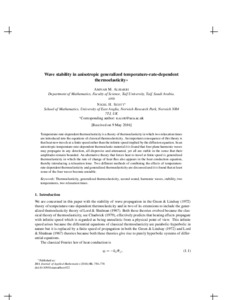# Wave stability in anisotropic generalized temperature-rate-dependent thermoelasticity

Alharbi, Amnah and Scott, Nigel H. (2016) Wave stability in anisotropic generalized temperature-rate-dependent thermoelasticity. IMA Journal of Applied Mathematics, 81 (5). pp. 750-778. ISSN 0272-4960Preview PDF (Accepted manuscript) - Accepted Version Download (3MB) | Preview

## Abstract

Temperature-rate-dependent thermoelasticity is a theory of thermoelasticity in which two relaxation times are introduced into the equations of classical thermoelasticity. An important consequence of this theory is that heat now travels at a finite speed rather than the infinite speed implied by the diffusion equation. In an anisotropic temperature-rate-dependent thermoelastic material, it is found that four plane harmonic waves may propagate in any direction, all dispersive and attenuated, yet all are stable in the sense that their amplitudes remain bounded. An alternative theory that forces heat to travel at finite speed is generalized thermoelasticity in which the rate of change of heat flux also appears in the heat conduction equation, thereby introducing a relaxation time. Two different methods of combining the effects of temperature-rate-dependent thermoelasticity and generalized thermoelasticity are discussed, and it is found that at least some of the four waves become unstable.

Item Type: Article thermoelasticity,gyrowaterbag model,generalized thermoelasticity,second sound,harmonic waves,stability,two temperatures,two relaxation times Faculty of Science > School of Mathematics Faculty of Science > Research Groups > Fluid and Solid Mechanics http://imamat.oxfordjournals.org/lookup/... Pure Connector 06 Oct 2016 10:00 22 Oct 2022 01:39 https://ueaeprints.uea.ac.uk/id/eprint/60763 10.1093/imamat/hxw022View Item